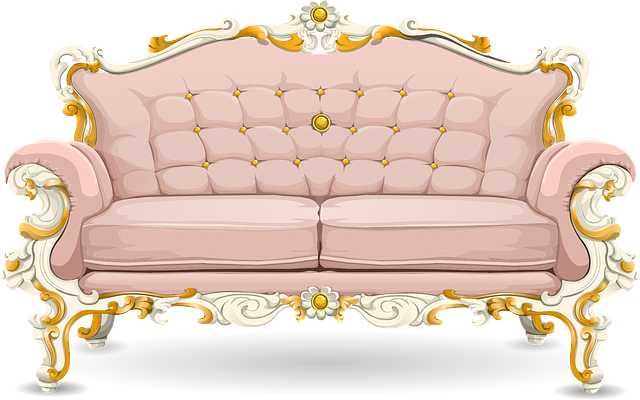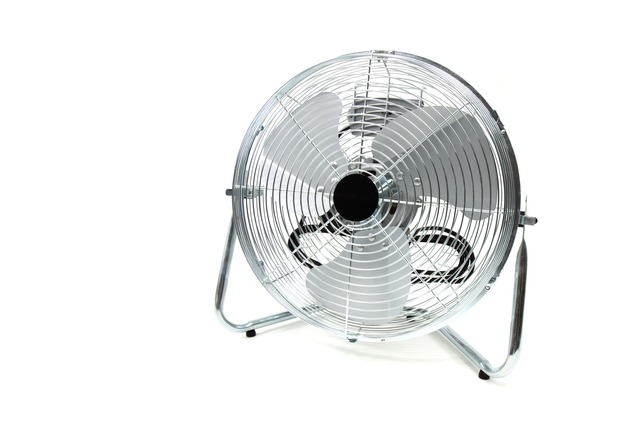## MTH213 Foundations of Elementary Math 3

### Fun with Geometry.Notes on Geometry
Spring 2020

#### Chapter 10 Geometric Figures

##### § 10.4 Symmetric Figures
• Reflection Symmetry or Line Symmetry• Rotational Symmetry
• center of rotation
• angle of rotation
• Examples:• Reflectional Symmetry for Space Figures• Rotational Symmetry for Space Figures• Relationships between reflection symmetries and rotational symmetries
Here we have 3 lines of symmetry and 120 degree rotational symmetry##### Math Activity: Pattern Block Symmetries

Purpose: Explore lines of symmetry and rotational symmetry.

Materials: Pattern blocks, paper, color.

1. Toy Theater Pattern Blocks, dragging feels real
2. Math Learning Center Pattern Blocks, nice protractor

Directions: First, identify the symmetries of each pattern block, then use the pattern blocks to create various other symmetric figures.

Part 1: Identify the symmetries of each pattern block below. Draw sketches and/or write descriptions for each.

Block Lines of symmetry rotational symmetryPart 2: Be more creative and create new figures from the pattern blocks.

Figure and Name Lines of symmetry Rotational symmetry
1.
2.
3.